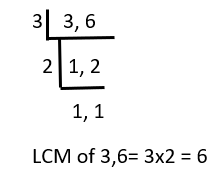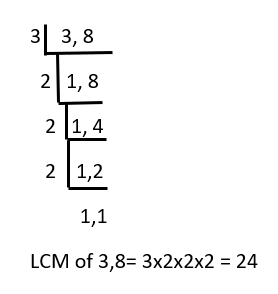GeeksforGeeks App
Open AppBrowser
Continue

# How to Add Fractions with Negative Numbers?

A fraction is a numeric value that defines as part of a whole. It is derived from the word ‘fractio’ means to break. It is used to solve daily life problems like dividing food, supplies, currency, etc. A number that is in the form of a/b can be called a fraction. Where a, b are any numbers. For example 2/3, 12/4, etc. Rational, Irrational numbers which are of form a/b can be considered as fractions.

Part of Fraction

Fraction consist of two parts:

• Denominator: The lowest part of the fraction is known as the denominator. It represents in which how many parts the given whole number will be divided. For example, 2/3, here 3 is known as denominator.
• Numerator: The upper part of the fraction is known as the numerator. It represents in which how many sections of the fraction. For example, 2/3, here 3 is known as numerator

Type of Fraction

Following are the type of fractions:

• Proper Fraction: Proper fractions are those fractions in which the numerator is always less than its denominator. For example, 5/16, 1/4, etc.
• Improper Fraction: Improper fractions are those fractions in which the numerator is always more than or equal to its denominator. For example, 5/2, 11/4, etc.
• Unit Fraction: Unit fractions are those fractions that have only 1 at the numerator. For example, 1/2, 1/14, etc.
• Mixed Fraction: Mixed fractions are those fractions that contain the mixture of whole and proper fractions. For example,, etc.
• Equivalent Fraction: Equivalent fractions are those fractions that contain the same values. For example, 2/9 x 2/2 = 4/18.
• Like Fraction: Like fractions are those fractions that contain the same denominators. For example, 2/8, 4/8, etc.
• Unlike Fraction: Unlike fractions are those fractions that contain different denominators. For example, 2/9, 8/13, etc.

### Add fractions with Negative Numbers

As we know addition is the basic operation of mathematics. It is used to find the sum of two positive or negative numbers. We can also add fractions with the same or different denominators. We are allowed also allowed to add fractions with negative numbers. Some rules we need to remember when we perform addition or subtraction between any positive or negative numbers. Those are mentioned below-

Rule 1: When two positive numbers are multiplied we get positive result.

(+) x (+) = +

Example: 5×2=10

Rule 2: When two negative numbers/symbols are multiplied we get positive result.

(-) x (-) = +

Example: (-7)×(-2)= 14

Here two negative symbols are cancelled to each other.

Rule 3: When a positive and negative integers are multiplied then resultant number will be negative.

(-) x (+) =

Example: (-7) x 2 = -14

So we need to consider these rules while performing the addition between fractions and negative numbers.

Steps to add fractions with negative numbers:

We have a fraction a/b and a negative number -c. Now we add them using the following steps:

Step 1: Convert their symbols according to the above rule. Here, a/b + (-c) = a/b – c

Step 2: a/b – c can also written as a/b -c/1

Step 3: Now take the LCM on b and 1

Step 4: Now the final equation is (a – c)/b and solve this equation to get the final result.

### Sample Questions

Question 1: (1/2) + (-1) = ?

Solution:

Here we have + operation before a negative number. According to rule-3 when we multiply + with – we get negative symbol.

(1/2) + (-1) will be converted to (1/2) – 1

This (1/2) – 1 can be rewritten into (1/2) – (1/1)

LCM of 2 denominators 2,1 is 2.

(1/2) – (1/1) = (1 – 2)/2

= -1/2

= -0.5

Question 2: -(1/2) + (-1) = ?

Solution:

Here we have + operation before a negative number. According to rule-3 when we multiply + with – we get negative symbol.

-(1/2) + (-1) will be converted to -(1/2) – 1

This -(1/2) – 1 can be rewritten into (-1/2) – (1/1)

LCM of 2 denominators 2,1 is 2.

(-1/2) – (1/1) = (-1 – 2)/2

= -3/2

= -1.5

Question 3: -(1/3) + (3/6) = ?

Solution:

LCM of 2 denominators 3, 6 is 6.(-1/3) + (3/6) = (-2 + 3)/6

= 1/6

Question 4: -(1/3) + (3/8) = ?

Solution:

LCM of 2 denominators 3,8 is 24.(-1/3) + (3/8) = ( (-1 x 8) + (3 x 3) )/24

=(-8 + 9) /24

= 1/24

Question 5: -(1/3) + (-2) = ?

Solution:

Here we have + operation before a negative number. According to rule-3 when we multiply + with – we get negative symbol.

(-1/3) + (-2) will be converted to (-1/3)-(2)

This -(1/3) – 2 can be rewritten into (-1/3) – (2/1)

LCM of 2 denominators 3, 1 is 3.

(-1/3) – (2/1) = (-1 – 2)/3

= -3/3

= -1

My Personal Notes arrow_drop_up
Related Tutorials